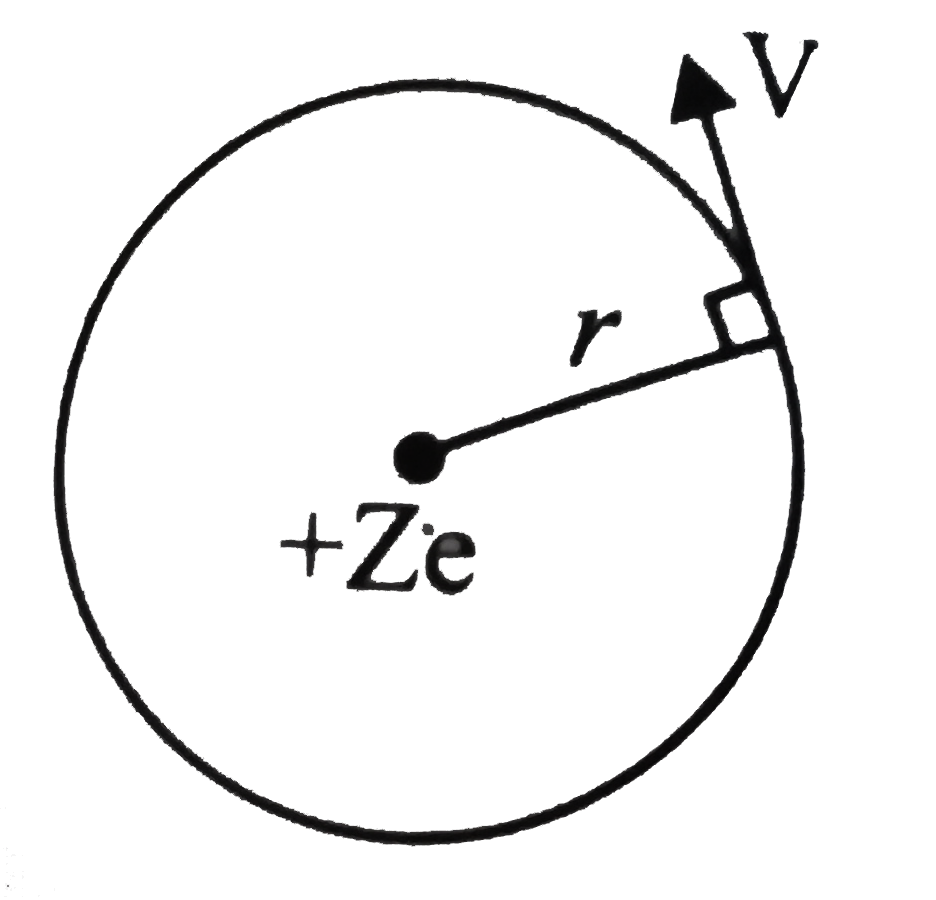# Determine the frequency of revalution of an electron in the second Bohr orbit in hydrogen atomKhareedo DN Pro and dekho sari videos bina kisi ad ki rukaavat ke!

##### Updated On: 27-06-2022

Text Solution

Solution
The frequency of revolation of an electron is given byfrequency = 1Time period
Time period = Total distance converd in one revolationvelocity
=2πrv
:. Frequency= v2πr
Calculate the velocity (v2) and radius (r2) of the electron in the second Bohr orbit in H atom
Z=1 for H atom
using rn=0.529n2ZÅ
We get
r2=0.529×1010(2)21m=1.12×1010m
vn=2.18×106ms1
v2=2.18×106(1/2)=1.09×106ms1
Frequency=v22πr2=1.09×1062(π)(2.12×1010)
v=8.18×1014Hz